•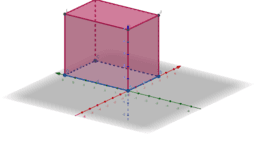Rectangular Prism 1

Activity

•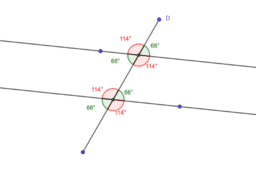Seeing parallel lines cut by a transversal APS

Activity

•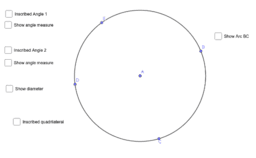Inscribed Angles and Inscribed Quadrilateral APS

Activity

•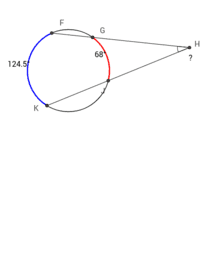Practice solving for arcs and angles in a circle APS

Activity

•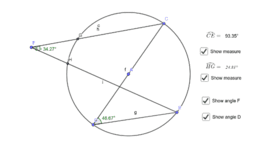Exploring Inscribed & Circumscribed Angles APS

Activity

•APS Geometry Unit 1 Book

Book

•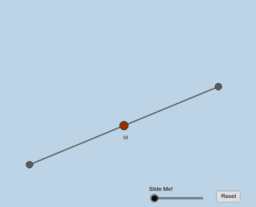Point / Midpoint / Segment Definition APS

Activity

•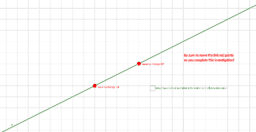Parallel Lines Definition APS

Activity

•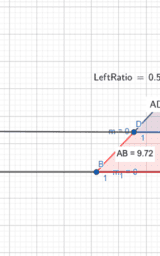Triangle Proportionality (Nested Triangles w/vertex 3rd side

Activity

•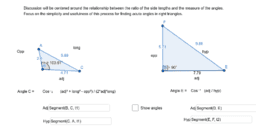Why are right triangles special? (finding angles) APS

Activity

•Exploring Rotations (Intervals of 90 degrees only) APS

Activity

•APS Geometry Unit 3

Book

•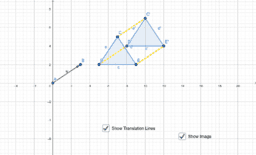Represent Translations

Activity

•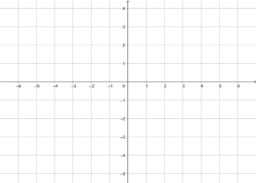Graphing Polygons

Activity

•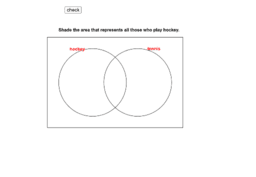Venn Diagrams Unit 7

Book

•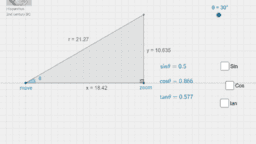Sine Cosine and Tangent

Activity

•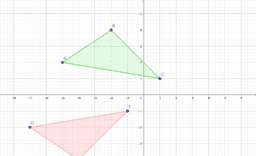Proving Congruence

Activity

•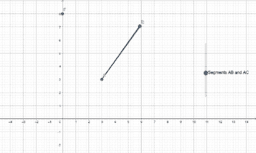Radii of a circle and Pythagorean Theorem

Activity

•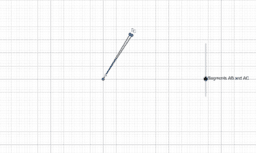Activity

•Select point D and notice the relationship between the angles as you move it around

Activity

•Select point D and notice the relationship between the angles as you move it around

Activity

•Select point D and notice the relationship between the angles as you move it around

Activity

•